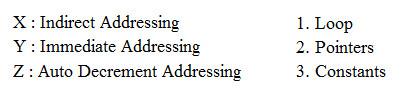# ISRO Scientist or Engineer Computer Science May 2017

Instructions

For the following questions answer them individually

Question 11

# Given the following statements S1 : Every context-sensitive language L is recursive S2 : There exists a recursive language that is not context-sensitive Which statements are true?Question 12

# Which one of the following is FALSE?Question 13

# In some programming languages, an identifier is permitted to be a letter followed by any number of letters or digits. If L and D denotes the set of letters and digit respectively. Which of the following expression defines an identifier?Question 14

# The recurrence relation that arises in relation with the complexity of binary search isQuestion 15

# Which one of the following in-place sorting algorithms needs the minimum number of swaps?Question 16

# Given two statements (i) Insertion of an element should be done at the last node in a circular list (ii) Deletion of an element should be done at the last node of the circular listQuestion 17

# Which of the following data structure is useful in traversing a given graph by breadth first search?Question 18

# How many $$128 \times 8$$ bit RAMs are required to design $$32K \times32$$ bit RAM?Question 19

# The most appropriate matching for the following pairs :Question 20

# Which interrupt in 8085 Microprocessor is unmaskable?OR